CAT  >  Sequence and Series questions

# Sequence and Series questions Notes | Study Quantitative Aptitude (Quant) - CAT

## Document Description: Sequence and Series questions for CAT 2022 is part of Progressions (Sequences & Series) for Quantitative Aptitude (Quant) preparation. The notes and questions for Sequence and Series questions have been prepared according to the CAT exam syllabus. Information about Sequence and Series questions covers topics like and Sequence and Series questions Example, for CAT 2022 Exam. Find important definitions, questions, notes, meanings, examples, exercises and tests below for Sequence and Series questions.

Introduction of Sequence and Series questions in English is available as part of our Quantitative Aptitude (Quant) for CAT & Sequence and Series questions in Hindi for Quantitative Aptitude (Quant) course. Download more important topics related with Progressions (Sequences & Series), notes, lectures and mock test series for CAT Exam by signing up for free. CAT: Sequence and Series questions Notes | Study Quantitative Aptitude (Quant) - CAT
 1 Crore+ students have signed up on EduRev. Have you?

Question 1: Find the following sum>
1/(22 –1) +1/(42 –1) + 1/(62 –1) + …. +1/(202 –1)
(a) 9/10
(b) 10/11
(c) 19/21
(d) 10/21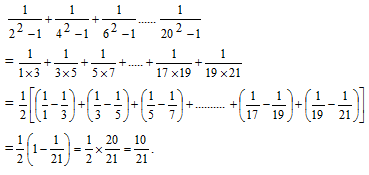Question 2: Two men X and Y started working for a certain company at similar jobs on January 1, 1950. X asked for an initial salary of Rs. 300 with an annual increment of Rs. 30. Y asked for an initial salary of Rs. 200 with a rise of Rs. 15 every six months. Assume that the arrangements remained unaltered till December 31, 1959. Salary is paid on the last day of the month. What is the total amount paid to them as salary during the period?
(a) Rs. 93,300
(b) Rs. 93,200
(c) Rs. 93,100
(d) None of these

The salary of X in the first year is Rs 300 and then it increases Rs 30 annually.

Hence, the total salary of X is given by:

X = 12 x (300 + 330 + 360 + 390 + 420 + 450 + 480 + 510 + 540 + 570)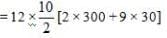[∵ sum of AP]

= 60 x 870 = Rs. 52,200

Similarly, the initial salary of Y was Rs 200 and it increased by Rs 15 every six months. So. the total salary of Y is given by

Y = 6 x [200+215 +230 + 245 +260 ........20 terms]

= 6 x 10 x [2 x 200-19 x 15]    [sumofA.P.]

= 60 x [400 + 285] = Rs. 41.100

Hence, the total salary-paid = 52200 + 41100 = Rs 93300.

Question 3: Let S denote the infinite sum 2 + 5x + 9x2 + 14x3 + 20x4 + …, where   | | < 1, then S equals
(a) {(2-x)/(1-x)3}
(b) {(2-x)/(1+x)3}
(c) {(2+x)/(1-x)3}
(d) {(2+x)/(1+x)3}

We will solve this question by using the given options.

In the first option we have,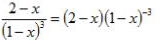Using the expansion for (1 - x)-3 = 1 + 3x + 6x2 + 10x3 + .....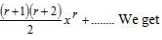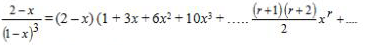= 2 + 5x+9x2 + 14x3 + ....

Which is equal to the given sum. Hence option 1 is the answer.

Question 4: The infinite sum 1 + (4/7) + (9/72) + (16/73) + (25/74) + …. equals
(a) 27/14
(b) 21/13
(c) 49/27
(d) 256/147

We have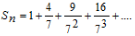.......(1)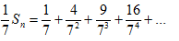.......(2)

Subtracting (2) from (1).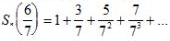.......(3)

Multiplying (3) by 1 7, we obtain equation 4: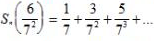.......(4)

Subtracting (4) from (3).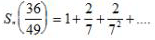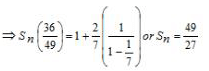Question 5: Consider the set S = (1, 2, 3, …, 1000}. How many arithmetic progressions can be formed from the elements of S that start with 1 and end with 1000 and have at least 3 elements?
(a) 3
(b) 4
(c) 6
(d) 7
(e) 8

Let number of terms in the arithmetic progression be n, then

1000 = 1 + (n–1) d

⇒ (n–1) d = 999

⇒ n – 1 = 999/d

Since n is an integer, so n – 1 is also an integer. This means that ‘d’ is a factor of 999.

Now 999 = 33 × 37. so the total factors of 999 are 4 × 2 = 8.

Out of these 8 factors one factor is 999 and we will reject it as in that case there will be only two terms in the

A.P. i.e. 1 and 1000, which is not possible.

Hence, ‘d’ can take 7 different values.

So, in total, 7 A.Ps. are possible.

The document Sequence and Series questions Notes | Study Quantitative Aptitude (Quant) - CAT is a part of the CAT Course Quantitative Aptitude (Quant).
All you need of CAT at this link: CAT

## Quantitative Aptitude (Quant)

162 videos|152 docs|131 tests
 Use Code STAYHOME200 and get INR 200 additional OFF

## Quantitative Aptitude (Quant)

162 videos|152 docs|131 tests

### How to Prepare for CAT

Read our guide to prepare for CAT which is created by Toppers & the best Teachers

Track your progress, build streaks, highlight & save important lessons and more!

,

,

,

,

,

,

,

,

,

,

,

,

,

,

,

,

,

,

,

,

,

;